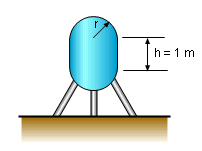Ch 3. Derivatives II Multimedia Engineering Math Higher OrderDerivatives RelatedRates Differential Newton'sMethod
 Chapter 1. Limits 2. Derivatives I 3. Derivatives II 4. Mean Value 5. Curve Sketching 6. Integrals 7. Inverse Functions 8. Integration Tech. 9. Integrate App. 10. Parametric Eqs. 11. Polar Coord. 12. Series Appendix Basic Math Units Search eBooks Dynamics Fluids Math Mechanics Statics Thermodynamics Author(s): Hengzhong Wen Chean Chin Ngo Meirong Huang Kurt Gramoll ©Kurt GramollMATHEMATICS - CASE STUDY IntroductionVessel Anson needs to design a high pressure vessel with a volume of 1.5 m3 for AlphaRx Company. The vessel consists of a cylindrical main section, 1 m high, and two hemispherical at each end.What should be the radius of both the two hemispherical ends and cylindrical middle? What is known: The volume of the vessel, v, is 1.5 m3. The height of the cylindrical part, h, is 1 m. Questions What is the radius, r, of the vessel? Approach The radius of both hemispherical ends and cylindrical middle are the same. The total volume needs to be determined in terms of r.